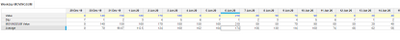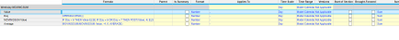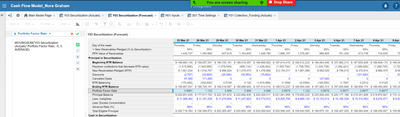# Finding the averageHi,

Need help creating a formula that pick's up the moving average for a number within 6 business days.

Example) The amount for a line item over the past Weekdays were 1, 4, 6, 8, 9. I want to forecast this by finding the average of those days.

Thank you

Tagged:

•The trick her is to manipulate the value for the days so that you replace the weekend value with the first two values of the moving 5 day average.

Therefore, as the moving average progresses the function includes the weekends but the values for Tues and Weds are posted forward into Sat and Sun so they are included in the movingsum.Create a new line which I have called, 'Movingsum Value', and using WEEKDAYS to distinguish which day of the week post forward 4 days if the weekday is 6 or 7. This has the effect of replacing Sat and Sun with the values from Tue and Wed.

You can then use the MOVINGSUM function as standard to calculate a moving average.•Have you tried using MOVINGSUM? Contrary to the name, it can also be used to calculate the moving average if you indicate the aggregation method.

You can see more on the formula here: MOVINGSUM

•Yes, we are using the Moving sum, but not getting back the correct average, I believe because it is picking up weekends (which we don't want it to do). This is the current formula we are using...

MOVINGSUM('Y03 Securitization (Actuals)'.Portfolio Factor Rate, -5, 0, AVERAGE)

we are trying to pick up the previous M, T, W, Th, F

••Hello @neg177 ,

You could achieve it still using the movingsum. But do not use the parameter Average.

Sum and divide by the count.

Using if condition, sum only the weekdays (skip the weekends).

XX-Y03 Securitization (Actuals) = if weekend then 0 else Y03 Securitization (Actuals)

For eg, if the current day is monday, your moving sum should be

Average = MOVINGSUM('XX-Y03 Securitization (Actuals)'.Portfolio Factor Rate, -6, 0, SUM) / 6

But you divide it by 6, so you will skip the weekend and calculate your average.

Hope it helps.

Thanks

Arun

•Hi,

Thank you it worked out great.